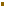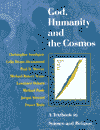### The Schrödinger Wave Equation

The fundamental equation of quantum mechanics is the Schrödinger Wave Equation - so-called because it takes a mathematical form characteristic of classical wave equations. However, the equation does not refer to physical waves but rather to probabilities, e.g. the probability of finding an electron in one location rather than another. The final outcome may be determinate (an electron in a particular location), but the probability distribution of the possible outcomes has the mathematical form of a wave. This peculiar feature of a very successful equation has led to the intractable problem of how we should interpret the theory that emerged from the quantum revolution. See Schrödinger’s Cat and the meaning of quantum theory.

Further discussion of the Schrödinger Wave Equation can be found in John Polkinghorne’s largely non-technical book The Quantum World.

Email link | Feedback | Contributed by: Dr. Christopher Southgate
Source: God, Humanity and the Cosmos  (T&T Clark, 1999)

### Quantum Physics and TheologyThe Schrödinger Wave Equation

#### Source:

 Dr. Lawrence Osborn and in God, Humanity and the Cosmos. Published by T&T Clark.